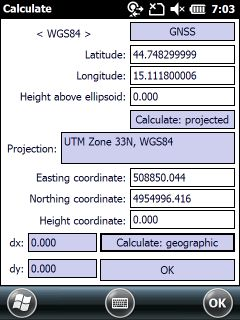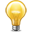﻿ Projections and Datums > The Calculate dialog box

# The Calculate panel

The Calculate panel calculates differences between two projections based on one reference point with WGS84 coordinates. This panel can be accessed on the Select Projection panel.

The Calculate panelThe Calculate panel contains the following controls:

GPS  - Takes over the current GPS coordinates to the reference position.

Latitude: Enter the Latitude coordinate of the reference point in WGS84.

Longitude: Enter the Longitude coordinate of the reference point in WGS84.

Height above ellipsoid: Height above the ellipsoid.

Calculate: projected  - Calculates the projected coordinates by using geographical coordinates

Projection: Shows the current projection.

Easting coordinate: Indicates the easting coordinate of the WGS84 reference point in the current projection.

Northing coordinate: Indicates the northing coordinate of the WGS84 reference point  in the current projection.

Height coordinate: Indicates the height of the WGS84 reference point in the current projection.

Calculate: geographic  - Calculates the geographical coordinates by using projected coordinates

dx: Indicates the horizontal difference between the current and the previously selected projection to the same reference point (with WGS84 coordinates).

dy: Indicates the vertical difference between the current and the previously selected projection to the same reference point (with WGS84 coordinates).

OK  - Closes the Calculates panel to select an other projection on the Select projection panel.The default geoid undulation file can be used in the calculations on the calculate dialog if it is exists in the \BIN directory in the Mobile version (SD Card) or in the installation directory in the Desktop version.